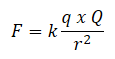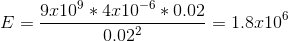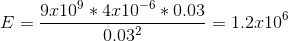•••••••••••••••••••••••••••••••••••••••••••••••••••••••••••••••••••••••••••••••••••• Home /Exam Details (QP Included)>Main Exam>Optional Subject-Science Group|Exam Details (QP Included)>Main Exam>Optional Subject-Medical Group>Physics / Dipole Field – Physics Notes – For W.B.C.S. Examination.
•## Dipole Field – Physics Notes – For W.B.C.S. Examination.

Dipole fields, or an electric field between two charges of the same but opposite strength, work in a similar way to the poles on the rock. Since positive charges have a field going out of them (pushing) and negative charges have a field going towards them (pulling) they create a current going from the positive charge to the negative charge.Continue Reading Dipole Field – Physics Notes – For W.B.C.S. Examination.

Charges are coming and going to all directions on both charges, and with a single point charge, these forces will be straight lines. But these lines curve towards the other charges when we have a dipole.

Let’s look at the positive charge, the charges would start going straight out from the positive charge, but would begin to curve inwards, towards the negative charge.

#### The X-axis in the Dipole Field

We can draw an x and y-axes in the dipole field, with the y-axis coming exactly halfway between the two charges and the x-axis going straight from one charge to the other. At any point along these axes, we can calculate the strength of the dipole field.

We know that the ‘rock’ will move towards the negative charge. We can calculate the force felt by that ‘rock’ by using
Coulomb’s law, where the electric strength equals Coulomb’s constants times the two charges, divided by the distance between the two charges squared. The force from each charge can be calculated and added together to find the total force felt by that point (or the ‘rock’).Let’s look back at our rock example, if your friend is the positive charge (pushing) and you are the negative charge (pulling) then the purple circle can be the rock, in-between both of you.##### Example Equation

Let’s look at a dipole field with the two charges having a strength of 4 microcoulombs. The two charges are 5 cm away from each other. What would be the electric field strength at a point 2 cm away from the positive charge (3 cm away from the negative charge)? We already know it will move towards the negative charge, and that both forces are pushing it in that direction, so now we just need to calculate the strength.

E=?

q= 4uC = 4×10 -6 C

k= 9.0 x 10 9 N*m 2/C 2

r(positive)= 2 cm = 0.02 m

r(negative) = 3 cm = 0.03 m